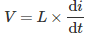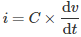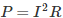# MCQs on Electric Circuits

##### Page 3 of 63. Go to page 1 2 3 4 5 6 7 8 9 10 11 12 13 14 15 16 17 18 19 20 21 22 23 24 25 26 27 28 29 30 31 32 33 34 35 36 37 38 39 40 41 42 43 44 45 46 47 48 49 50 51 52 53 54 55 56 57 58 59 60 61 62 63
01․ Inductor does not allow the sudden change of
current
voltage
power
None of the above

Voltage developed across an inductor is expressed bySudden change of current means di will change at dt → 0 (dt tends to zero), so sudden change of current means infinite voltage. Therefore it is not possible.

02․ Capacitor does not allow the sudden change of
current
voltage
power
None of the above

The expression of current through a capacitor is1. For sudden change of voltage infinite current is required. But practically it is not possible.
2. Practical capacitor circuit has finite value of time constant

03․ Internal resistance of ideal voltage source is
zero
infinite
finite
100 ohms

Ideal voltage source delivers energy at specified voltage, which is independent of current delivered. So voltage drop is zero and then resistance is zero. Its internal series resistance must be minimum to delivered maximum voltage.

04․ Internal resistance of ideal current source is
zero
infinite
finite
100 ohms

Ideal current source delivers energy at specified current, which is independent on voltage across the load. The internal resistance must become very high to deliver maximum current.

05․ Nodal analysis can be applied for
planar networks.
non planar networks.
both planar and non planar networks.
neither planar and non planar networks.

Nodal analysis is applied at different nodes of an electric network. So there is no problem with the planar or non planar circuits, so this is applicable for both planar and non planar networks.

06․ Mesh analysis is applicable for
planar networks.
non planar networks.
both planar and non planar networks.
neither planar and non planar networks..

Mesh analysis is nothing but loop analysis, this is applied for different loops in the network and the network should be planar network. Non planar networks can't form loop equation.

07․ Super position theorem is not applicable for
current calculations.
voltage calculations.
power calculations.
None of the above.

Super position theorem is used for calculation of voltage and currents only. It is not applicable for power calculations. Superposition theorem states that, in any linear bidirectional circuit having more than one independent source, the response in any one of the branches equal to algebraic sum of responses caused by individual sources, while the rest of the sources are replaced by its internal resistance. Therefore superposition theorem is only applicable for linear quantities. Whereas power is a non linear quantity.So superposition theorem is not applicable for power calculations.

08․ To apply reciprocity theorem response to excitation ratio is
Ohm.
Mho.
No units.
Either Ohm or Mho.

Conditions to apply reciprocity theorem are
1. Response to excitation ratio should be either Ohm or Mho.
2. Network should consists of one independent source only.
3. When a network have many dependent sources, reciprocity theorem can't be applied.

09․ Which quantity should be measured by the voltmeter ?
Current
Voltage
Power
Speed

Voltmeter is a high resistance device used for measuring potential difference or voltage. It must be connected in parallel for measurement.

10․ Which quantity consists of a unit 1KWh ?
Energy
Time
Power
Charge

1 kWh is the amount of energy used by a 1 kW (1000 watt) resistance for 1 hour. Energy = Power × Time.

<<<12345>>>